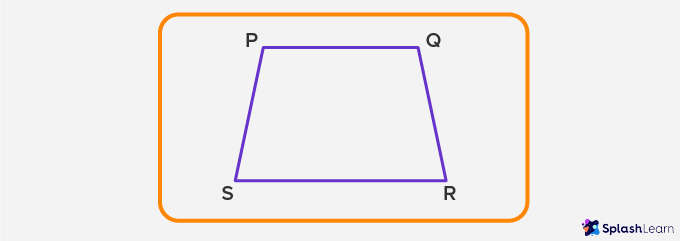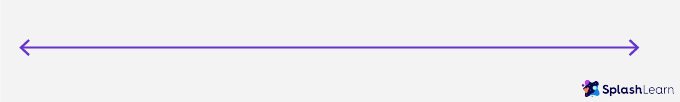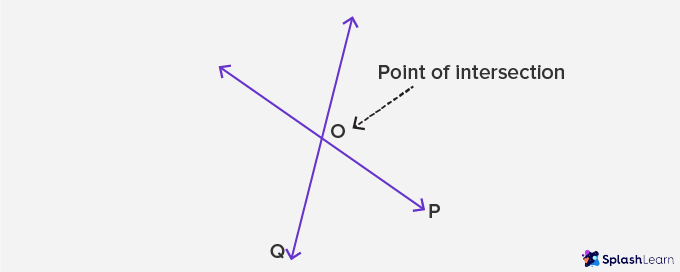# Line – Definition, Types of Line, Examples, Practice Problems

Home » Math-Vocabluary » Line – Definition, Types of Line, Examples, Practice Problems

## What is a Line?

In geometry, a line is a straight one-dimensional figure that does not have a thickness, and it extends endlessly in both directions.

Diagram A represents a line. It does not have any endpoint. The two arrows at each end signify that the line extends endlessly and is unending in both directions.  The length of a line cannot be measured.

Diagram A: Line

l in the above diagram represents a line.

In simple terms, when a set of collinear points are connected in a one-dimensional plane, it is called a line

## What is a Line Segment?

A line segment, which is part of a line, has two defined endpoints.

Diagram B represents a line segment with two endpoints, A and B. A line segment can be measured, whereas a line cannot. The length of the line segment is the distance between the two endpoints, A and B.

Diagram B: Line Segment

• Let’s draw a line

To draw a line, take a pencil, a ruler, and a book or a piece of paper.

1. Place the ruler on the paper and hold it firmly.
1. With the help of the pencil, draw a line along the horizontal edge of the ruler.
1.  Label the line as either l or m

Come, let’s sing along!
Length of the table at which you dine,
Ray of the sun in the morning that shine,
The straight tubes and pipes in a pipeline,
We can find in them – straight geometry lines!

•        Closely-related concepts to line

To better understand the concept of a line, let’s look at the following diagram along with the explanation mentioned below.

•        Examples of a Line

Did you know we are surrounded by objects that represent straight lines? They are all around us. Let’s look at some real-life objects as examples for your reference.

1. A pencil or a pen is a representation of a straight line.
1. A straight line is drawn on a piece of paper either horizontally or vertically with a pencil.
1. The edge of a table represents a straight line.
1. A scale or a ruler, a tool to measure length, is a straight line.

•       History of Line

Euclid, a Greek mathematician – fondly known as the Father of Geometry – introduced the concept of a line. He defined it as “a breadthless length.”

## Different Types of Line

Now Let’s understand the different types of lines.

• Straight Line

A line is a set of collinear points with no curves and extends limitlessly in opposite directions is called a Straight line.

• Curved Line

A line is a set of collinear points which has a curvature or bend in its formation is called a Curved Line.

• Oblique Line

An oblique is a line that is diagonal or slanting. However, it is not perpendicular, parallel, vertical, or horizontal.

• Horizontal Line

A horizontal line is a straight line that starts from either left to right or right to left. In simple terms, a horizontal line is also known as a sleeping line.

A particular line at which the earth’s surface and the sky appear to meet is called the horizon. Anything parallel to the horizon is known to be horizontal.

• Vertical Line

A vertical line is a straight line that starts from the top to bottom or bottom to top.  In simple terms, a vertical line is also known as a standing line.

Moreover, vertical is the opposite of horizontal. Anything that makes a 90-degree angle or a right angle with the horizontal line or the horizon is called vertical.

Moreover, there are also certain variations of the types of lines. It occurs when more than one line is placed in the same dimension.

• Parallel Line

A pair of two lines that are on the same plane and the distance between them is equal and remains constant. When such two lines do not intersect with each other, they are known as parallel lines

• Intersecting Line

On the contrary, when two or more pairs of lines are on the same plane and intersect each other at one given point, they are known as intersecting lines

• Transversal Line

A transversal line is a line that passes through two or more parallel or non-parallel lines at a given point. The parallel lines and the transversal line must be on the same plane.

• Perpendicular Line

When two lines on the same plane intersect each other and form a 90° angle at the point of intersection, they are known to be perpendicular lines

Let’s get to know a few interesting facts about lines.

1. A line is made up of an infinite number of points.
1. A line does not have width or thickness. It can only extend in length limitlessly.
1. When we place two points at each end of the line, it forms a line segment. Additionally, when we put one point at one end and an arrow at the other end, it forms a ray.
1. If three or more points are placed on the same line, they are called collinear points.
1. Euclid, in his book Elements, which is one of the most influential books ever written, has referred to the term line several times.

## Solved Examples On Line

1. Which of the following diagrams is a line?

Answer: The second diagram is a line as the two endpoints represent and includes an arrow, which extends endlessly in opposite directions.

1. Identify the diagram and explain if it is a pair of parallel lines or perpendicular lines?

Answer: The above diagram shows perpendicular lines as both the lines intersect at one point and form an angle of 90° at the intersection.

1. Which of the following diagrams shows a pair of parallel lines?

Answer: The 2nd diagram shows a pair of parallel lines, as both are on the same dimensional plane and do not intersect each other.

## Practice Problems On Line

1

### Choose the correct pair of parallel lines from the given options:PS II PQ
PS II QR
PQ II SR
QR II SR
CorrectIncorrect
Correct answer is: PQ II SR
The correct answer is PQ II SR.
2

### Identify the diagramA line
A ray
A line segment
An angle
CorrectIncorrect
The correct answer is a line as it extends indefinitely in both directions.
3

### What type are the below pair of lines known asTransversal lines
Parallel lines
Horizontal Lines
Intersecting Lines
CorrectIncorrect
The correct answer is intersecting lines, as the lines intersect each other at a point.

## Frequently Asked Questions On Line

In geometry, a ray is defined as a one-dimensional figure with a fixed starting point. However, it has no endpoint.

A line segment is part of a line that has 2 specified endpoints in both directions and has a definite length.

Unlike a line, which does not have any endpoints, a line segment has two endpoints.

No, a line only has length. It does not have width or thickness.

A line does not have any endpoint. For reference, a ray has one endpoint, and a segment has two endpoints.

A line can be referred to or labeled using two letters at the ends of the line, usually in uppercase, such as A and B. A line is generally referred to with a single letter, such as ‘l’ or ‘m’, usually in lowercase.

•        Tips to master the concept of Line

We at SplashLearn have curated games, worksheets, and courses for children to identify types of lines and courses to differentiate between lines, line segments, rays, and angles.

It is highly beneficial for children to learn through games and worksheets customized in an interactive and engaging format. It is exciting for them, as their brains tend to grasp visuals more easily and memorize them quickly.
SplashLearn offers personalized grade and standard-based education material. Visit www.splashlearn.com to know more.